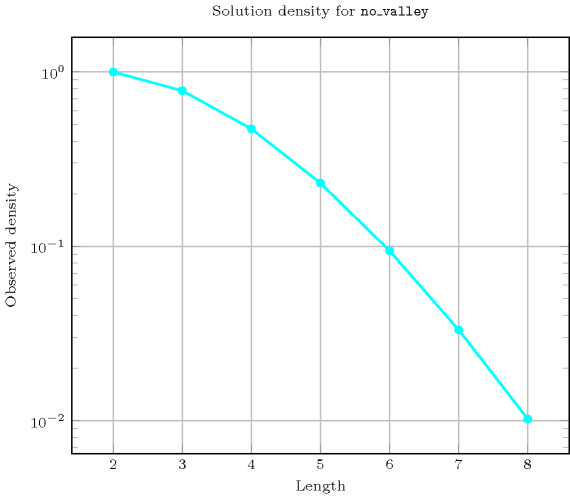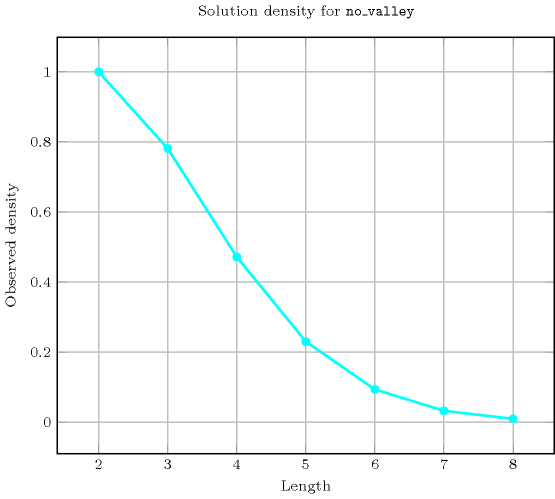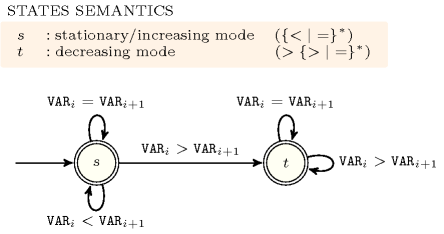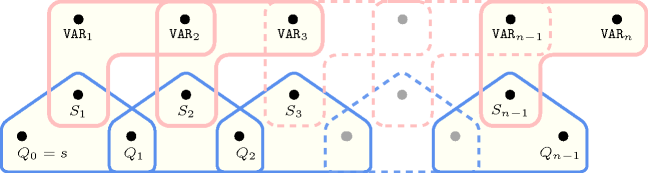## 5.279. no_valley

Origin

Derived from $\mathrm{𝚟𝚊𝚕𝚕𝚎𝚢}$.

Constraint

$\mathrm{𝚗𝚘}_\mathrm{𝚟𝚊𝚕𝚕𝚎𝚢}\left(\mathrm{𝚅𝙰𝚁𝙸𝙰𝙱𝙻𝙴𝚂}\right)$

Argument
 $\mathrm{𝚅𝙰𝚁𝙸𝙰𝙱𝙻𝙴𝚂}$ $\mathrm{𝚌𝚘𝚕𝚕𝚎𝚌𝚝𝚒𝚘𝚗}\left(\mathrm{𝚟𝚊𝚛}-\mathrm{𝚍𝚟𝚊𝚛}\right)$
Restrictions
 $|\mathrm{𝚅𝙰𝚁𝙸𝙰𝙱𝙻𝙴𝚂}|>0$ $\mathrm{𝚛𝚎𝚚𝚞𝚒𝚛𝚎𝚍}$$\left(\mathrm{𝚅𝙰𝚁𝙸𝙰𝙱𝙻𝙴𝚂},\mathrm{𝚟𝚊𝚛}\right)$
Purpose

A variable ${V}_{k}$ $\left(1 of the sequence of variables $\mathrm{𝚅𝙰𝚁𝙸𝙰𝙱𝙻𝙴𝚂}={V}_{1},\cdots ,{V}_{m}$ is a valley if and only if there exists an $i$ $\left(1 such that ${V}_{i-1}>{V}_{i}$ and ${V}_{i}={V}_{i+1}=\cdots ={V}_{k}$ and ${V}_{k}<{V}_{k+1}$. The total number of valleys of the sequence of variables $\mathrm{𝚅𝙰𝚁𝙸𝙰𝙱𝙻𝙴𝚂}$ is equal to 0.

Example
$\left(〈1,1,4,8,8,2〉\right)$

The $\mathrm{𝚗𝚘}_\mathrm{𝚟𝚊𝚕𝚕𝚎𝚢}$ constraint holds since the sequence $114882$ does not contain any valley.

##### Figure 5.279.1. Illustration of the Example slot: a sequence of five variables ${V}_{1}$, ${V}_{2}$, ${V}_{3}$, ${V}_{4}$, ${V}_{5}$, ${V}_{6}$ respectively fixed to values 1, 1, 4, 8, 8, 2 without any valleyTypical
 $|\mathrm{𝚅𝙰𝚁𝙸𝙰𝙱𝙻𝙴𝚂}|>3$ $\mathrm{𝚛𝚊𝚗𝚐𝚎}$$\left(\mathrm{𝚅𝙰𝚁𝙸𝙰𝙱𝙻𝙴𝚂}.\mathrm{𝚟𝚊𝚛}\right)>1$
Symmetries
• Items of $\mathrm{𝚅𝙰𝚁𝙸𝙰𝙱𝙻𝙴𝚂}$ can be reversed.

• One and the same constant can be added to the $\mathrm{𝚟𝚊𝚛}$ attribute of all items of $\mathrm{𝚅𝙰𝚁𝙸𝙰𝙱𝙻𝙴𝚂}$.

Arg. properties

Contractible wrt. $\mathrm{𝚅𝙰𝚁𝙸𝙰𝙱𝙻𝙴𝚂}$.

Counting
 Length ($n$) 2 3 4 5 6 7 8 Solutions 9 50 295 1792 11088 69498 439791

Number of solutions for $\mathrm{𝚗𝚘}_\mathrm{𝚟𝚊𝚕𝚕𝚎𝚢}$: domains $0..n$generalisation: $\mathrm{𝚟𝚊𝚕𝚕𝚎𝚢}$ (introduce a $\mathrm{𝚟𝚊𝚛𝚒𝚊𝚋𝚕𝚎}$ counting the number of valleys).
Figure 5.279.2 depicts the automaton associated with the $\mathrm{𝚗𝚘}_\mathrm{𝚟𝚊𝚕𝚕𝚎𝚢}$ constraint. To each pair of consecutive variables $\left({\mathrm{𝚅𝙰𝚁}}_{i},{\mathrm{𝚅𝙰𝚁}}_{i+1}\right)$ of the collection $\mathrm{𝚅𝙰𝚁𝙸𝙰𝙱𝙻𝙴𝚂}$ corresponds a signature variable ${S}_{i}$. The following signature constraint links ${\mathrm{𝚅𝙰𝚁}}_{i}$, ${\mathrm{𝚅𝙰𝚁}}_{i+1}$ and ${S}_{i}$: $\left({\mathrm{𝚅𝙰𝚁}}_{i}<{\mathrm{𝚅𝙰𝚁}}_{i+1}⇔{S}_{i}=0\right)\wedge \left({\mathrm{𝚅𝙰𝚁}}_{i}={\mathrm{𝚅𝙰𝚁}}_{i+1}⇔{S}_{i}=1\right)\wedge \left({\mathrm{𝚅𝙰𝚁}}_{i}>{\mathrm{𝚅𝙰𝚁}}_{i+1}⇔{S}_{i}=2\right)$.
##### Figure 5.279.2. Automaton of the $\mathrm{𝚗𝚘}_\mathrm{𝚟𝚊𝚕𝚕𝚎𝚢}$ constraint##### Figure 5.279.3. Hypergraph of the reformulation corresponding to the automaton of the $\mathrm{𝚗𝚘}_\mathrm{𝚟𝚊𝚕𝚕𝚎𝚢}$ constraint (since all states of the automaton are accepting there is no restriction on the last variable ${Q}_{n-1}$)